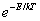# Physics 751 Homework #1

Due Friday September 5, 11:00 am.

1.         (a) Suppose the electron in a hydrogen atom is in the circular Bohr orbit with n = 30.  How many times per second does it go around?

(b) Suppose now the electron drops to the n = 29 state, emitting a single photon. What is the frequency of this photon, in cycles per second?

(c) Comment on the relation between your answers in (a), (b) above.  What would you guess the relation to be for n = 300?

2.         Rutherford established that all the positive charge in an atom was concentrated in a massive central nucleus by analyzing the scattering of α-particles, which are He nuclei.  He found that the numbers scattered as a function of angle of deflection were as predicted using a model of a point nucleus with the usual inverse-square repulsion. Would this be true if the α’s actually hit the nucleus?  Find (somewhere) the approximate size of the gold nucleus (he used gold foil) and deduce what minimum energy of the incoming α -particle would be expected to show deviation from the inverse-square law prediction of scattering.  Would the minimum energy be different if aluminum were used instead?

3.         The μ (muon) is a cousin of the electron, the only difference being its mass is 207 times greater.  The μ has a lifetime of about 2 microseconds. If a beam of μs is directed at a solid, the μs will go into orbit around nuclei. The Bohr atom, with a μ replacing the electron, is a useful model for picturing this.

(a) For a nucleus of charge Ze, how large is the n = 1 μ orbit compared with the electron orbit?

(b) What is the frequency of the photon emitted by the μ in the n = 2 to n = 1 transition?

(c) For the gold nucleus, the n = 1 μ orbit is inside the nucleus.  Find the frequency of the emitted photon for n = 2 to n = 1 in this case. (Hint: you’ll need the radius of the gold nucleus—but you found that for question 2.  Assume here that the positive charge is uniformly spread throughout the nucleus.)

4.         A helium atom is resting on a table top, held in place by the gravitational force. Use the uncertainty principle to estimate the uncertainty in the height of the atom above the table. (This is meant to be an order-of-magnitude question. Don’t worry about factors of 2, π etc.)

5.         Suppose the electron in a Bohr atom radiated energy as classically predicted for an accelerating charge. Approximately how long would the atom last?  (Find and use a simple formula for radiation from an accelerating charge.)

6.         If a one-dimensional classical simple harmonic oscillator having mass m and spring constant C at temperature T occupies all possible states over time with relative probability, show that the average value of its energy is kT, independent of m or C.